Written by Andrea Fields MHA, RDCS

### Aortic Stenosis: Breaking Down the Continuity Equation

The evaluation and screening of aortic stenosis is a routine calculation performed on all complete echocardiograms. The detailed evaluation involves multiple key parameters that make up the equation that determines the aortic valve area (AVA). With echo being the gold-standard to evaluating and diagnosing the severity of aortic stenosis, it is crucial we understand the methodology of the continuity equation and how the fluid dynamics play a key role in the calculation.

# Continuity Equation: AVA

The continuity equation is based on the conservation of mass… since the volume of blood cannot be ‘lost’ – this theory supports the concept that what flows in, must flow out! In other terms: the volume proximal to valve is the same as the volume downstream from the valve.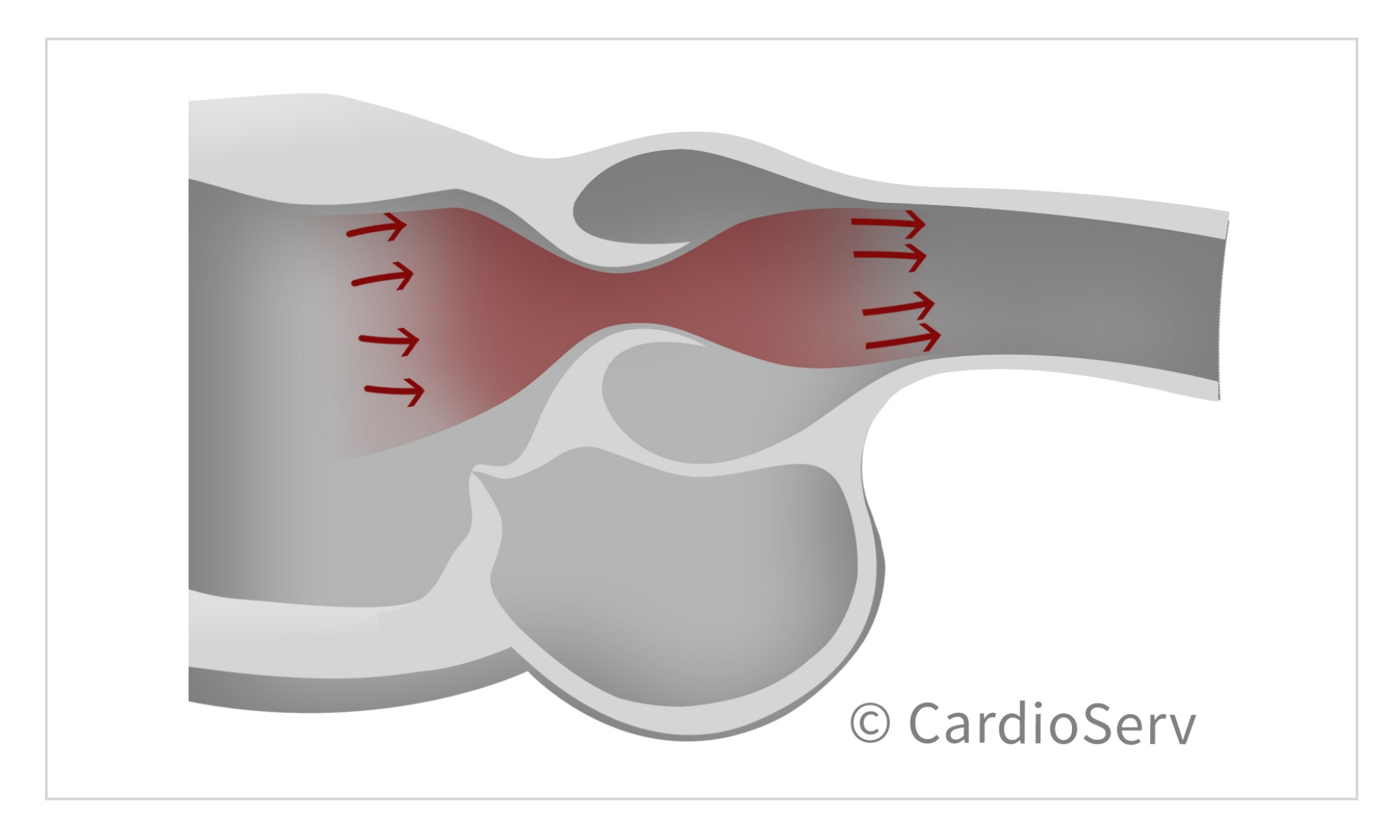It evaluates the highest gradients across the valve to determine the effective orifice area (EOA). Due to fluid dynamics, higher gradients of a stenotic valve will occur post-valve (downstream) at the narrowest portion of jet flow, this is the location of the EOA. The calculated EOA is smaller than the anatomical orifice area (AOA), which is the planimetry traced area. The EOA (continuity equation) is the primary predictor of clinical outcomes.The continuity equation involves 4 key elements:

1. LVOT CSA (A1)
2. LVOT VTI (V1)
3. AV CSA (A2)
4. AV Peak VTI (V2)The stroke volume through the LVOT is equal to the stroke volume through the aortic valve. We can obtain 3 of the 4 components by calculating the stroke volume of the LVOT and measuring the peak AV velocity (V2). This allows us to solve for the 4th component, which is the AVA!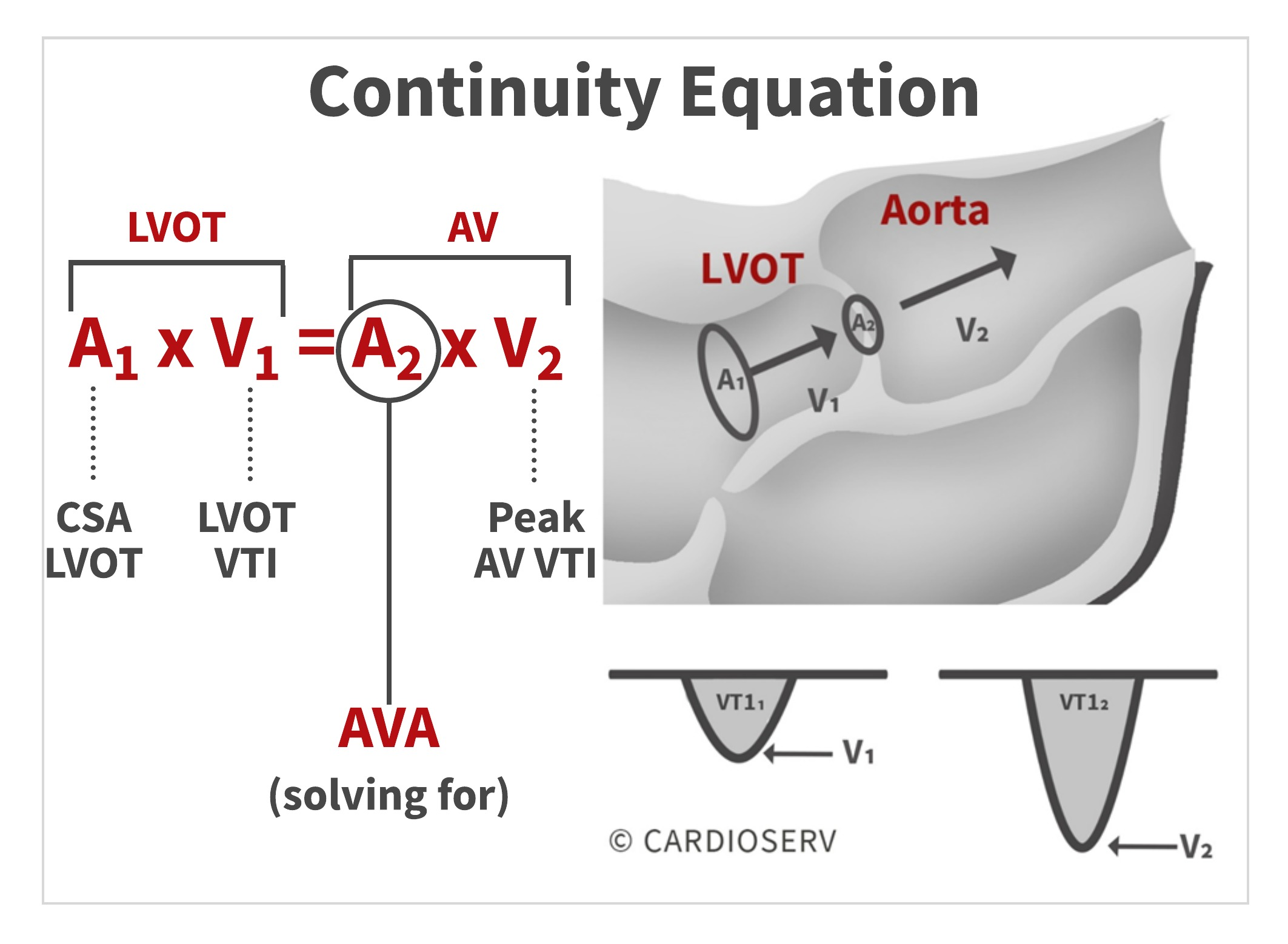By filling in echo-obtained calculations into the conservation of mass theory, we can calculate the continuity equation for the aortic valve area (AVA)!To calculate the AVA using the continuity equation, we need 3 parameters:

1. LVOT Diameter
2. LVOT Doppler VTI
3. AV Peak Doppler VTI

Let’s break down the equation to better understand the methodology behind it!

# Doppler Stroke Volume

First, let’s discuss how we calculate Doppler stroke volumes. Doppler stroke volume (SV) is the volume of blood that passes through a specific location during each beat [mL]. Since the continuity equation states there is equal SV of blood going in and coming out of an orifice, we need to calculate the SV of one of the locations.

We can calculate the SV of the LVOT by measuring the:

1. Cross-sectional Area
2. Velocity-time Integral (VTI)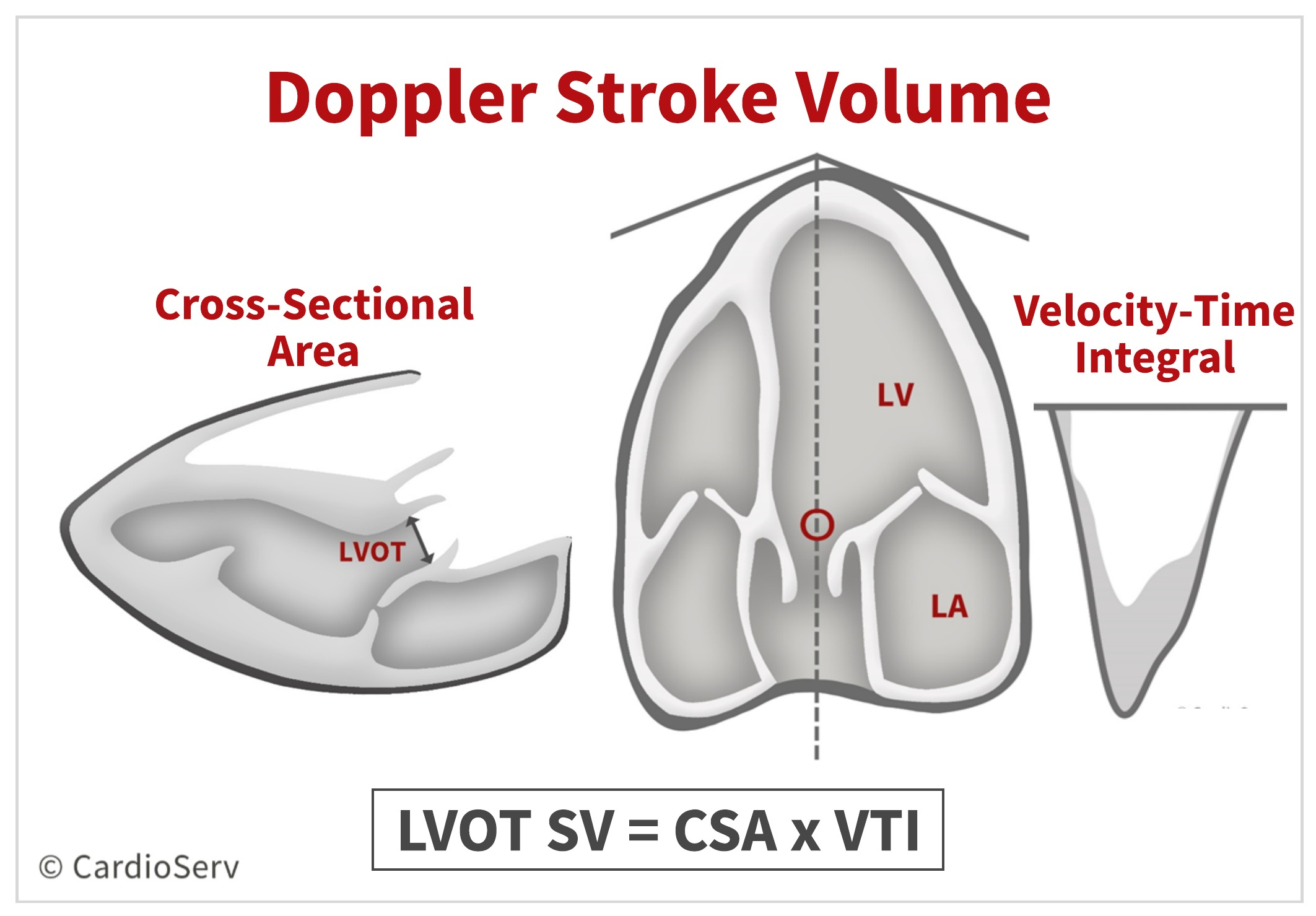# Part 1: LVOT Diameter

To determine the cross-sectional area (cm²), we need to measure the diameter (d) of the LVOT.

• Zoomed parasternal long axis view (PLAX)
• Mid-systole
• Inner-to-inner
• Parallel to aortic valve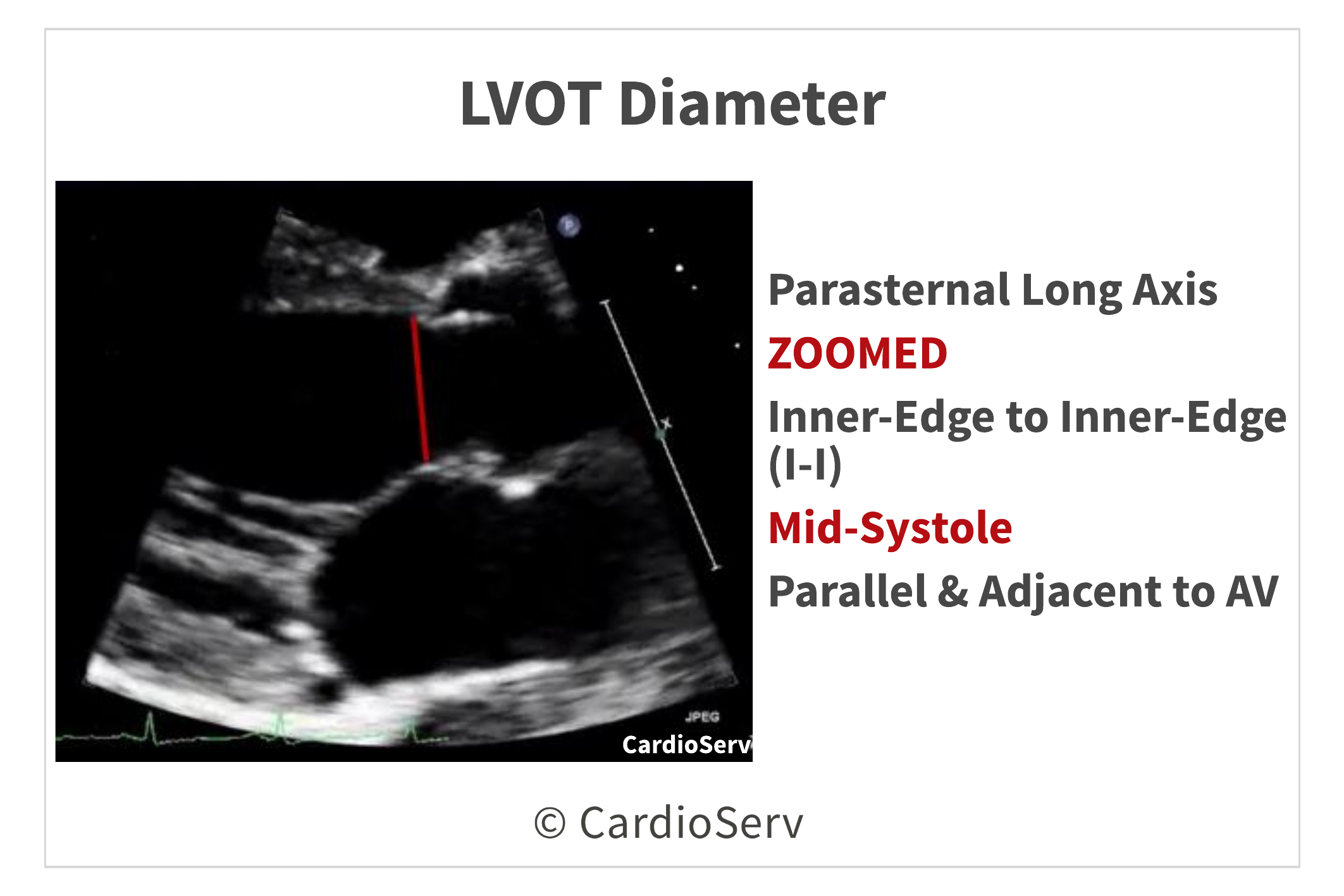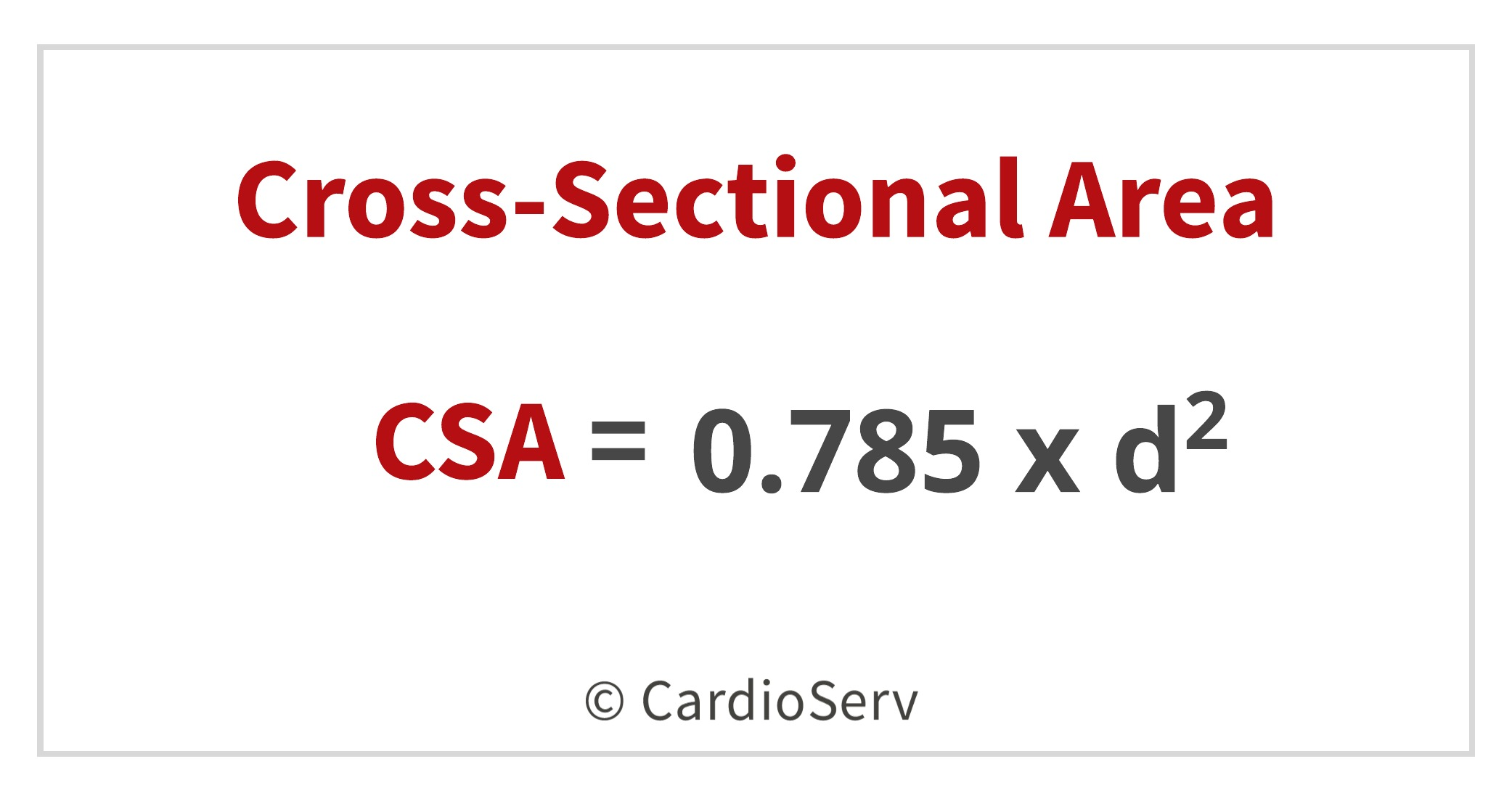# Part 2: LVOT Doppler VTI

The second part to calculating the stroke volume is to obtain a pulsed-wave (PW) Doppler of the LVOT.

• Apical 5 view
• Sample volume proximal to aortic valve
• Adjust sample volume to obtain laminar flow curve
• Tracing the VTI of the modal velocity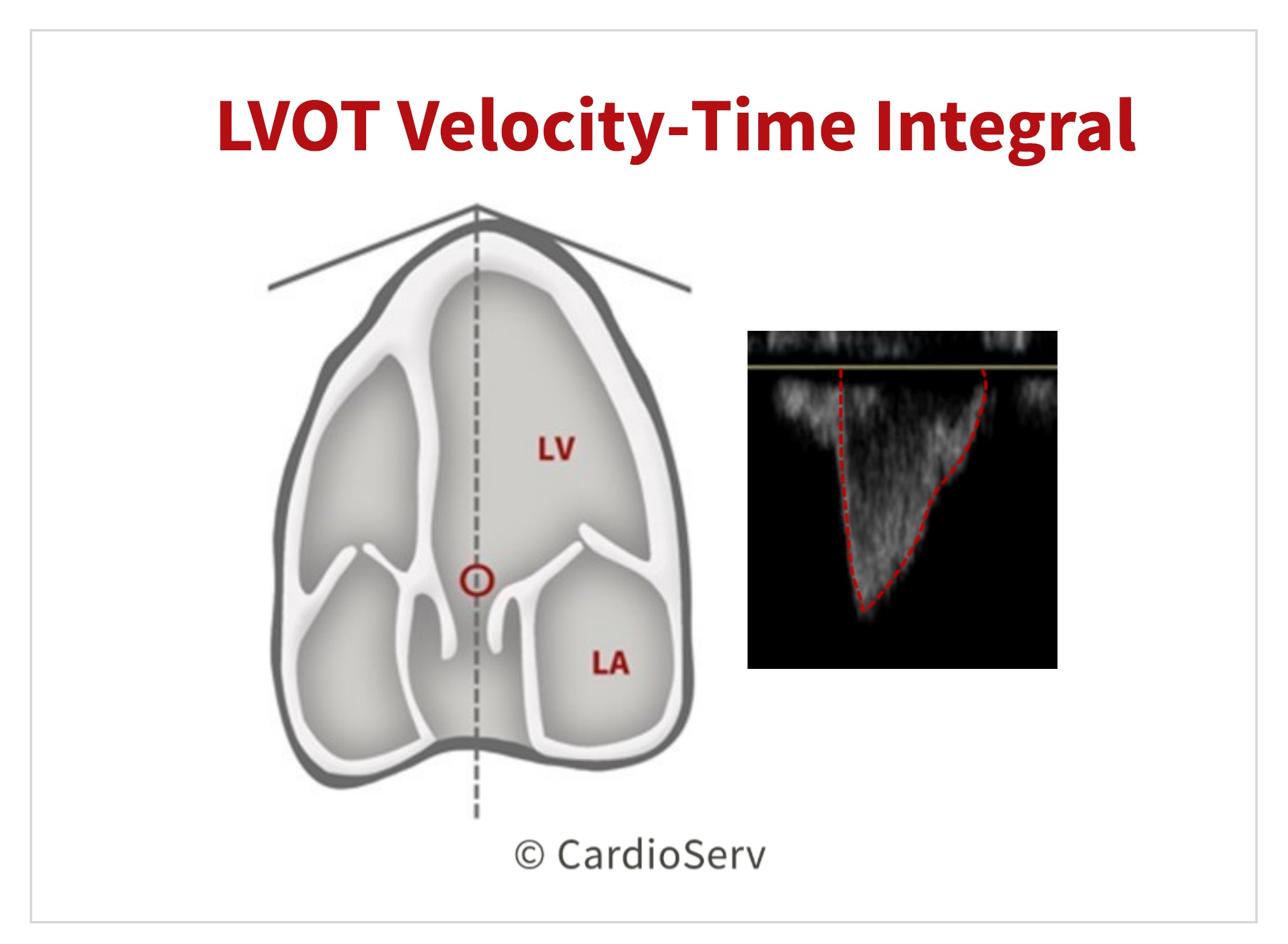## Doppler Stroke Volume Calculation

Now that we have the 2 components, we can calculate the Doppler SV of the LVOT!# Part 3: Peak Aortic Valve VTI

We have now obtained 2 of the 3 components needed for the continuity equation, we need the last part:

1. LVOT CSA – CHECK!
2. LVOT VTI – CHECK!
3. Peak AV Velocity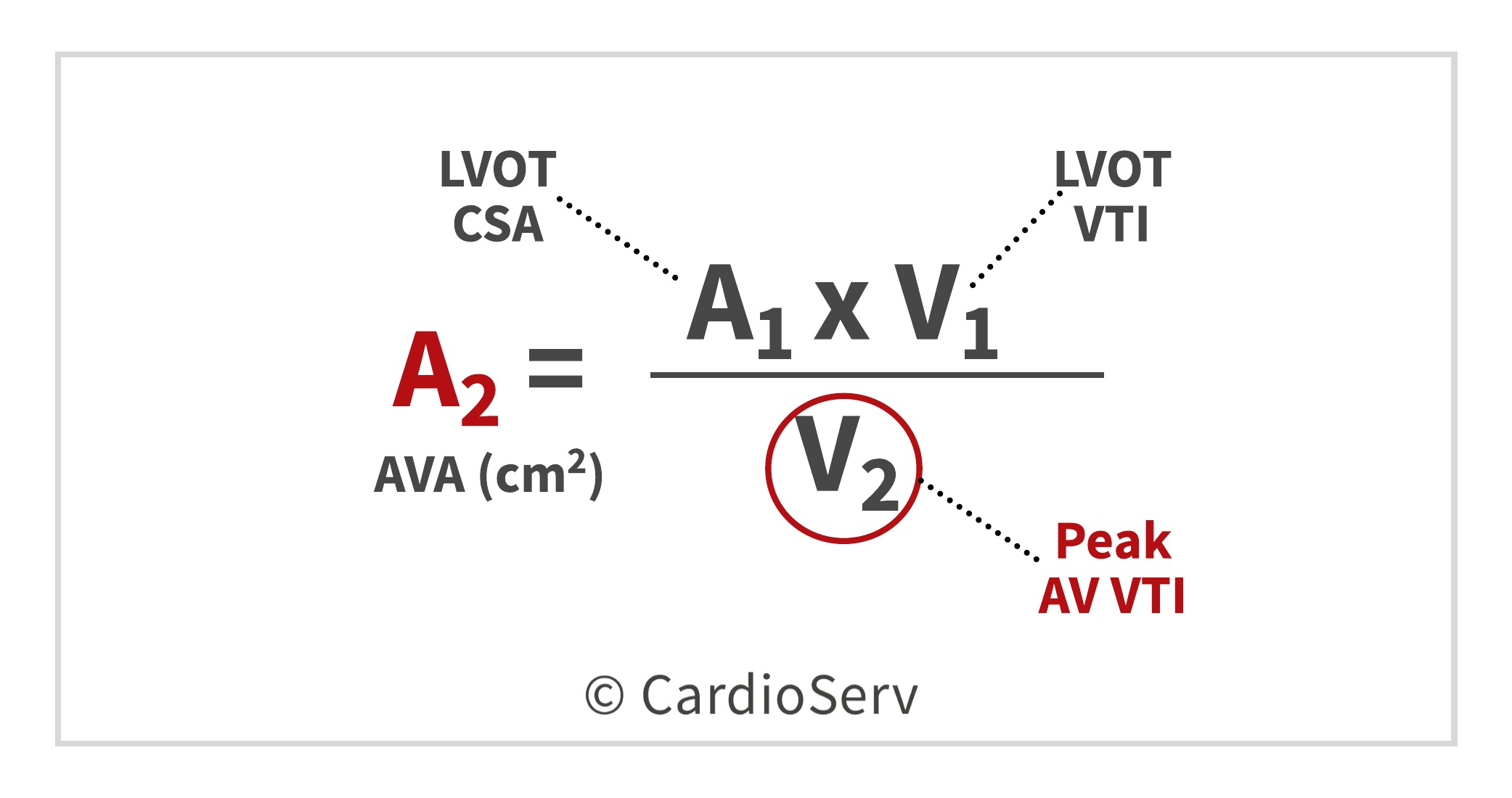## AV Peak Velocity

The 3rd component needed to calculate the AVA is the peak AV VTI! This antegrade systolic flow should be evaluated in multiple acoustic windows to determine the highest velocity.

• CW Doppler
• Maximum velocity at peak of dense velocity curve
• Ultrasound beam parallel to direction of flow
• Trace outer edge of dense signalReview our past blogs that cover tips and techniques for measuring and obtaining the aortic valve velocity!

# Continuity Equation: AVA

Finally we can calculate the AVA using the continuity equation after obtaining the 3 parameters: LVOT diameter, LVOT VTI & AV Peak VTI.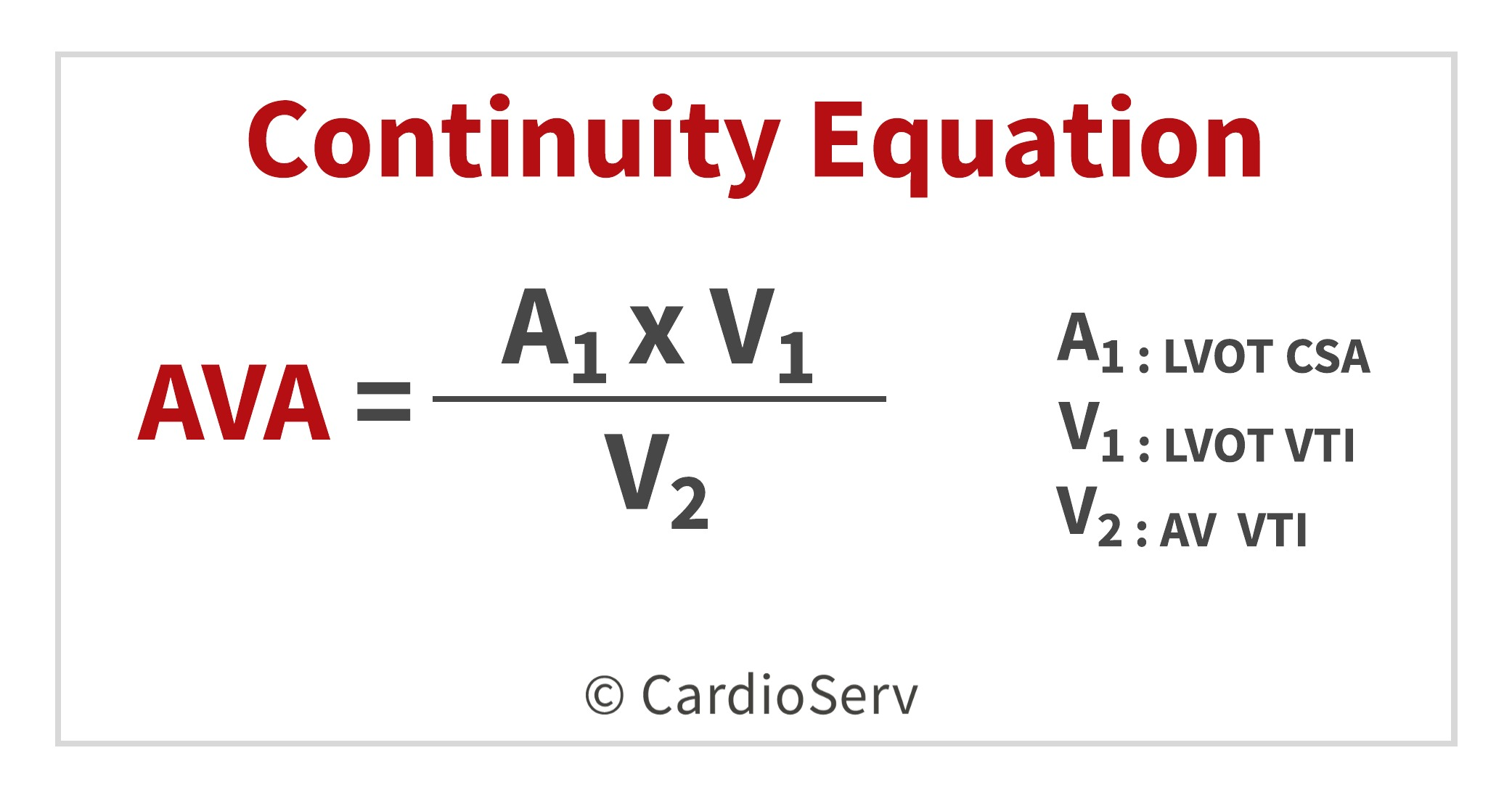The chart listed below are the cut-off ranges for determining the severity of stenosis using the continuity equation:## Continuity Equation Limitations

There are limitations to the continuity equation due to the multiple variables involved in the equation. The following are key limitations that should be taken into consideration when determining the AVA:

1. Variability in acquiring and measuring 3 components of equation.
2. Assumption of circular shape of LVOT, rather than an elliptical- which can underestimate the LVOT CSA.
3. Room for potential error when calculating the SV of LVOT, specifically in the PW Doppler obtained measurement. Factors such as subaortic membranes and aortic regurgitation can alter the assumed laminar flow profile and cause miscalculation.
4. Location placement of sample volume when obtaining the PW Doppler of LVOT is another potential place for error. If sample volume is placed outside the cross-sectional area of the LVOT, the Doppler can yield higher (closer to septum) or lower (closer to anterior mitral valve leaflet).

There are other theoretical concerns regarding the continuity equation as well:

1. Continuity equation measures the EOA instead of the anatomical orifice area (AOA). This can cause discrepancies when comparing echo to cath-obtained values. However there has been evidence & data that supports the use of continuity equation using the EOA over AOA, as primary predictor of clinical outcome.
2. Valve area change in regards to flow rate. When there is decreased LV ejection fraction resulting in ability for aortic leaflets to open properly, the EOA can calculate severe even though severe stenosis might not be present. Further investigation of flow rate should be performed to determine true severity of stenosis.

On a positive note- there are advantages to the continuity equation method too!

1. Validated in both clinical & experimental studies.
2. Continuity equation AVA found to be valuable parameter for prediction of clinical outcome & decision-making.
3. Measures effective orifice area (EOA)
4. Feasible to obtain in majority of patients

# Summary

The continuity equation is an important concept to understand and apply to our patients. Being able to comprehend the methodology and flow dynamics is essential to ensuring proper and correct methods when calculating the aortic valve area.Andrea Fields MHA, RDCS

References:

Lang, R. M., MD, Badano, L. P., MD, & Mor-Avi, V., PhD. (2015). Recommendations for Cardiac Chamber Quantification by Echocardiography in Adults: An Update from the American Society of Echocardiography and the European Association of Cardiovascular Imaging. JASE, 28(1), 1-53. Retrieved March 1, 2017, from http://asecho.org/wordpress/wp-content/uploads/2015/01/ChamberQuantification2015.pdf

# Our Services

## LET US KNOW WHAT YOU THINK...

Ferry Koevoets
Good day Andrea Nice article on the AVA calculation with the equation method and your explanation of the flow measurements. I cannot not always obtain the LVOT diameter on more difficult patients with calcified Aortic valves, this is where the continuity equation method becomes interesting!
Wazir. Khan
You seem to be a gifted teacher.looking forward to see more of your posts.
Sachin Swami
Good presentation
NABIL ATALLAH
can we send the patient with aortic stenosis depending on our ECHO doppler calculation of AVA , TO SURGERY WITHOUT CATH .
Cardioserv
Due to the prevalence of coronary artery disease in conjunction with coronary artery disease, it is recommended for the patient to have a cardiac cath prior to surgery. There are many articles on line from the European and American Heart Associations that you may find beneficial including the European Heart Journal, Volume 35, Issue 31.
Saeed
Very nice presentation ...thank u
RAJASEKAR PERIYASAMY
Impressive teaching and demonstration... Makes me involved